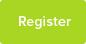# Understanding The Context Of Questions In Mathematics And Choosing Appropriate Method For Solving.

This piece gives an example understanding of context and appropriately choosing method to solve a mathematical problem.

Date : 24/03/2019### Author Information

Subject : Maths

As the examinations are approaching, I thought it may well be appropriate to point out the importance of carefully paying attention to context of questions.

Rather than talking in general terms, an example will make this piece short and quickly come to the point.

Take single variable quadratic expression using x as variable in Cartesian coordinate system. One way of finding maximum (or minimum) is by completing square. ;

Another way is to use the fact that the graph of value of the single variable quadratic expression against the variable x is of parabolic profile and parabolic profile is symmetric about the vertical line at x where the expression reaches maximum or minimum. So, finding the two x values where the expression becomes zero by equating the expression to zero, solve it as quadratic equation and then find the middle x value of those two x values where the expression becomes zero.

Finding minimum and maximum of single variable quadratic expression appeared as an examination question in different flavours over the years.

At one time, I believe examiners wanted to test the understanding how students are able to relate and apply quadratic expression to real word situation. ; ;

The examiners gave the scenario of height of a rocket above the ground level (h) from the time the rocket was launched by a quadratic expression in terms of time, t (t being the variable). The question was to find the maximum height the rocket could reach above the ground and the time at which could the rocket reach maximum height above the ground.

As an example, lets say h = 2 + 18t -1.5(t^2).

The completing square can be used to find the answers. ;

The second method will yield correct answers in numbers. ;

However, it is completely wrong application to the situation. ;

The reason is by saying h= 0, therefore ; 2 + 18t -1.5(t^2) =0, the solutions are for when height of the rocket above ground is zero.

Without stating the equation represents parabolic profile, symmetrical property of parabolic profile and maximum (or minimum) is reached at the midpoint of the points where h =0, the students had shown indeed incorrect understanding ; of how the equation relate to physical significance of rocket flight.

Yet, another method is to use differential calculus. The calculus method yields stationary points, where instantaneous rate of change is zero. ;

Whether the height of the rocket above the ground found by the second method is indeed maximum or not remains unanswered in both second and calculus method. Students need to show that indeed it is the maximum height. I will leave it as an exercise for students.

So, be watchful and careful about the context of questions and try to see what examiners are trying to aim so that students can appropriately choose method and provide reasoning for answers. ;

This resource was uploaded by: Kidner